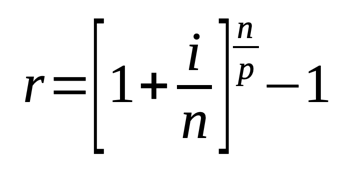# Amortized Loan Calculator

Share:

This calculator is used to calculate the Amortized Loan. You can use this calculator to calculate the periodic payment amount of loans like mortgages, housing loans, student loans, personal loans or auto loans.

Enter the required fields and press the Calculate Loan button to get the result.

*
*
\$
*
%
*
*
*
Years
Months
From
\$
\$
at the end
\$This calculator is used to calculate the amortized loan repayment amount for each payment period. It also calculates the total amount to be repaid, interest amount and the effective annual percentage rate. This calculator uses compounding of interest for interest calculation. It also has an option to include down payment, extra fee and balloon payment at the end of the loan term.

### Using Amortized Loan Calculator

1. Select the Currency of your choice.
2. Enter the Loan Amount amount (principal amount).
3. Enter the yearly compound Interest Rate in percentage.
4. Select the Compounding Period from the drop down.
5. Select the Payment Frequency from the drop down.
6. Enter the Loan Term either in years or months.
7. Optional Fields: If needed enter the Down Payment, Extra Fee and the Balloon Payment fields.
8. Finally, press the Calculate Loan button. The periodic payment amount, total interest amount, total payment amount, effective annual percentage rate (EAR), number of payments to do and the pay off date will be calculated and the result will be displayed.

## Amortized Loan

There are several types of loans like personal loan, mortgage, student loan, auto loan, small business loan, etc... Based on the repayment method it can be further classified as amortized loan, deferred payment loan or bond. Amortized loan is one in which a fixed payment is paid periodically till it matures.

### Formula To Find Amortized Loan Periodic Payments

This formula is used for calculating repayment of loan amount per period.##### Where:
• A = Repayment of loan amount per period
• P = Loan Amount
• r = Interest Rate per period
• n = Total number of payments or total number of periods

#### Formula To Find Interest Rate per Period##### Where:
• r = Interest rate per period
• i = Nominal annual interest rate
• n = Number of compounding periods / year
• p = Number of payment period / year